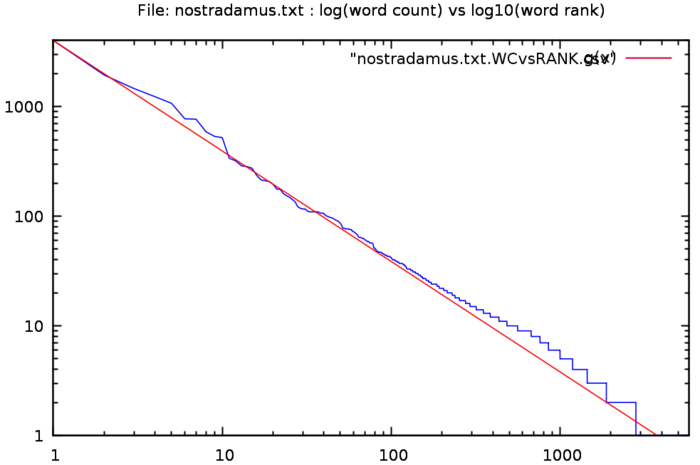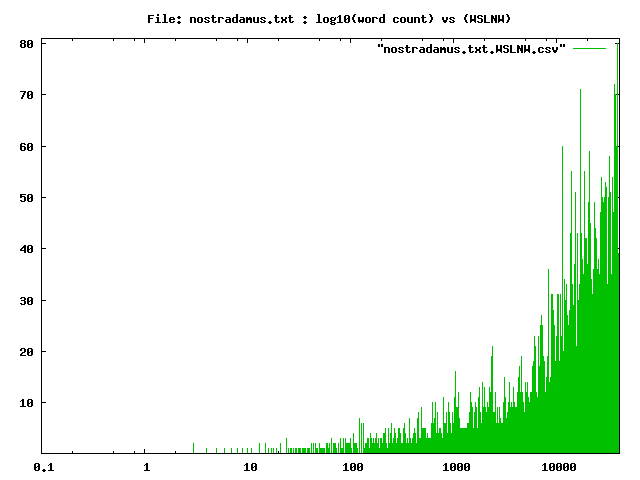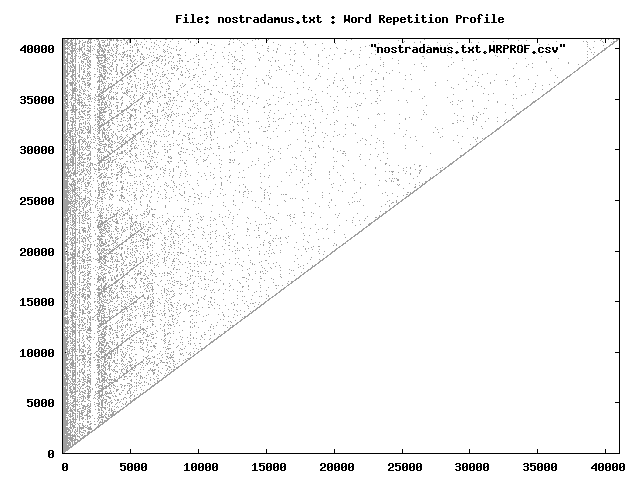Statistics on the text file nostradamus.txt, using the command:zwc -s -d -l -o outfiles -f nostradamus.txt The total word count is 41016 The unique words number 5818 The ratio unique/total is 14.1847 % The highest ranking 73 words make up 50.061 % of the total, OR 1.255 % of the unique words make up 50.061 % of the total volume The number of words used only once 3009 The ratio (words used only once)/total is 7.3362 % The average of the ratios between frequencies is 1.0016